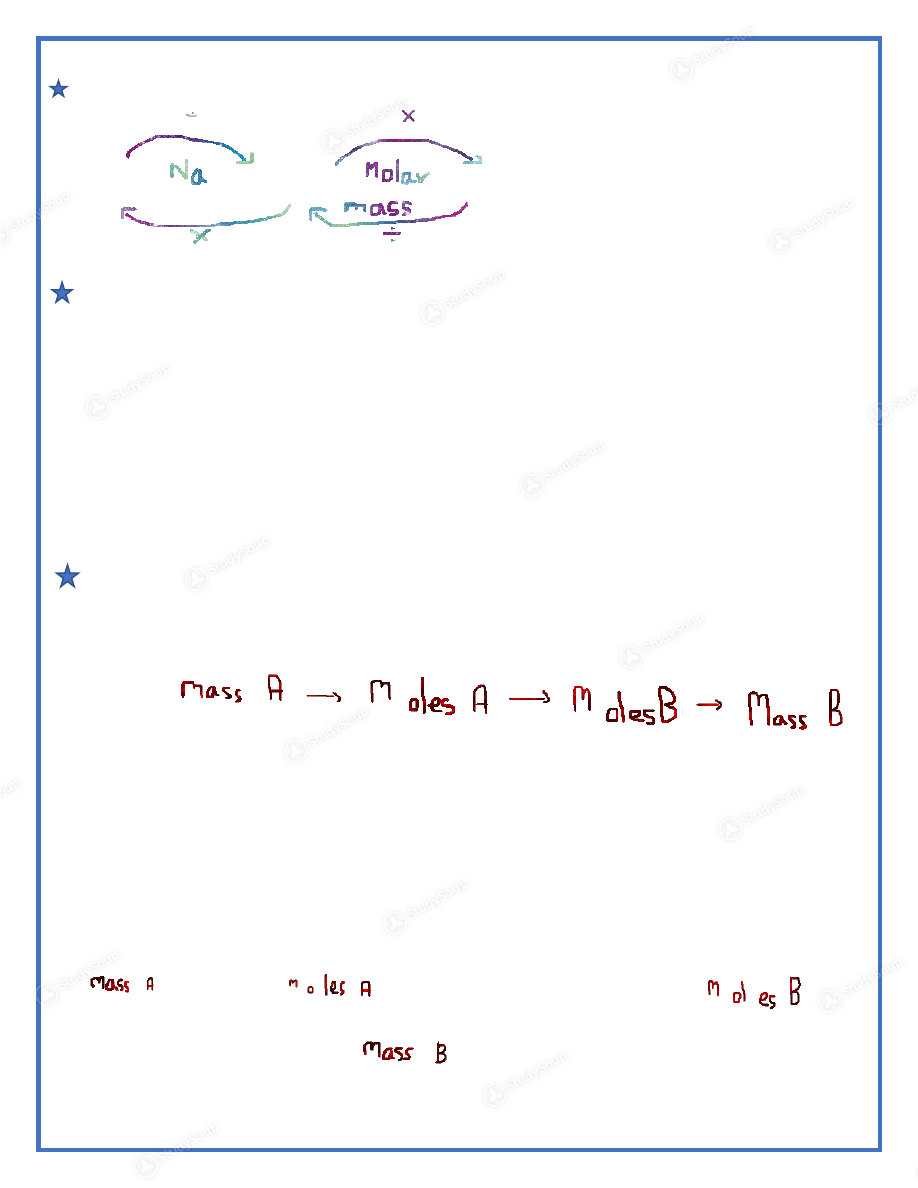Limited time offer 20% OFF StudySoup Subscription details

# UF - CHM 1025 - CHM Ch 6+7 Study Guide - Study Guide

### Created by: Philip Ambattu Elite Notetaker

> > > > UF - CHM 1025 - CHM Ch 6+7 Study Guide - Study Guide

UF - CHM 1025 - CHM Ch 6+7 Study Guide - Study Guide

##### Description: These notes cover whats going to be on our next exam. Ch.7 is incomplete because Roland will go over it on Tuesday.
0 5 3 2 Reviews
This preview shows pages 1 - 2 of a 6 page document. to view the rest of the contentCHM1025----Intro. To Chemistry Study Guide for Exam on Nov.1st         ©Philip Ambattu

Terms to know for the exam

1.  actual yield - the amount of product actually obtained in the laboratory from a reaction.
2.  endothermic reaction - a chemical change that absorbs heat.
3.  exothermic reaction - a chemical change that releases heat.
4.  heat - the energy that is transferred between objects due to a difference in their temperatures.
5.  law of conservation of energy - a law stating that energy can be converted from one form to another, or
transferred, but it cannot be created or destroyed.  6.  limiting reactant - the reactant that is completely used up in the reaction, therefore determines the # other  reactants that react and the products that should form.  7.  percent yield - the ratio of the amount of product actually formed in a reaction to what is predicted by  stoichiometry, multiplied by 100%.  8.  specific heat - a property that is a measure of the # of heat required to raise the temperature of 1 g of a  substance by 1°C.  9.  stoichiometry - the use of quantitative relationships between substances involved in a chemical reaction to  determine the amount of a reactant or product.  10. Theoretical yield: theoretical yield - the maximum amount of product that can be obtained in a chemical  reaction from known amounts of reactants; the amount of product calculated by assuming all the limiting
reactant is consumed.
11.  atomic radius - a measure of atomic size; the distance from the nucleus to the outer edge of the atom.
12.  aufbau principle - an approach to writing ground-state electron configurations of atoms by filling orbitals
that are lowest in energy first; means “building up” in German.  13. continuous spectrum - a spectrum in which all the visible wavelengths are present.
14. core electron - an electron in an atom that is not a valence electron; an inner electron.
15. electromagnetic radiation - a form of energy that can be described in terms of oscillating waves that move
through space at the speed of light; also called light or radiant energy.  16. electron configuration - a description of the distribution of electrons in atomic orbitals or sublevels
17. frequency - the number of wave cycles passing a stationary point in 1 second.
18. ground state - the state of lowest energy in an atom where the electrons occupy orbitals that are lowest in
energy.  19. hertz (Hz) - a unit of frequency equal to 1/s, or cycles per second.
20. Hund’s rule - a rule stating that electrons are distributed in a set of orbitals of identical energy in a way to
give the maximum number of unpaired electrons.  21. ionization energy - the minimum energy required to remove a valence electron from a gaseous atom / ion;  usually reported in kJ/mol.  22. isoelectronic - having the same number of electrons.
23. line spectrum - a spectrum produced by a substance in which only certain wavelengths are present.
24. orbital - a three-dimensional region in space around a nucleus where an electron is likely to be found.
25. Pauli exclusion principle - a principle stating that a maximum of two electrons can occupy an orbital,+ the
electrons must have opposite spin.  26. photon - the smallest packet of energy that describes the energy of a specific type of electromagnetic  radiation.  27. principal energy level - an energy level containing a set of electrons in orbitals of similar sizes.
28. valence electron - an electron in the highest principal energy level, the valence level.
29. wavelength - the distance between two corresponding points (such as the crests) on a wave.From previous lecture: Great Flow Chart to remember and use for conversions

Molecules                                 Moles                              Masses

Mole-Mole Conversions

2Al(s) + 6HNO
3  → 2Al(NO 3 ) 3  + 3H 2
First, always make sure the equation is balances, like it is above.

Problem: If 3 mol Al react completely, how many mol H2 will be produced?
Ans: Multiply the mol amount of Al by a fraction that has original number of moles of H2 and Al from
Equation in problem.
3 mol Al (3 mol H2/2 mol Al) = 9/2=4.5 mol H2.
Solution: 4.5 mol H
2

Mass-Mass Conversions

***The general formula to convert from a Mass A to a Mass B is as follows (as evidenced from flow chart):

Problem: 2 Na(s) + Cl
2  → 2NaCl(s)
If 9.0 g Na is there, how much Cl2 do I need to completely consume sodium?

Ans: (USE FORMULA ABOVE)
9.0 g Na/22.99g/mol = 0.391 mol Na →(0.391 mol Na x (1mol Cl
2 /2 mol Na))= .196 mol Cl 2

→ (.196 mol Cl
2  x 70.9 g/mol)= 13.9 g Cl 2

Solution: 13.9 g Cl

This is the end of the preview. Please to view the rest of the contentJoin more than 18,000+ college students at University of Florida who use StudySoup to get ahead
6 Pages 75 Views 60 Unlocks
• Notes, Study Guides, Flashcards + More!Join more than 18,000+ college students at University of Florida who use StudySoup to get ahead
##### Description: These notes cover whats going to be on our next exam. Ch.7 is incomplete because Roland will go over it on Tuesday.
6 Pages 75 Views 60 Unlocks
• Notes, Study Guides, Flashcards + More!
Get Full Access to UF - CHM 1025 - Study Guide - Midterm
×
Get Full Access to UF - CHM 1025 - Study Guide - Midterm

I don't want to reset my password

Need help? Contact support

Need an Account? Is not associated with an account
We're here to help